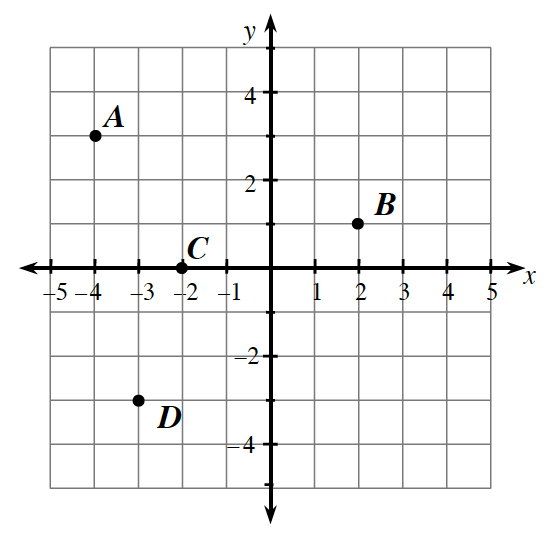### Home > CCA > Chapter A > Lesson A.1.2 > ProblemA-20

A-20.

Examine the graph at right. Then complete parts (a) and (b) below.1. In $\left(x, y\right)$ form, name the coordinates of points $A, B, C$, and $D$.

Find the change in $x$ and $y$ from the point $\left(0,0\right)$.

Point $A$ goes to the left $4$ spaces and up $3$ spaces.
How do you write that in $\left(x, y\right)$ form?

$A$ is $\left(−4,3\right)$. Now find points $B, C$, and $D$.

2. On graph paper, draw a set of axes like the ones shown at right. Then plot points $E\left(5, 2\right), F\left(−3, −1\right), G\left(0, −4\right)$, and $H\left(2, −3\right)$.

Remember that the horizontal axis is the $x$-axis, and the vertical axis is the $y$-axis.